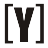# Amps (A) to Kilowatts (kW) Calculator

This Amps to Kilowatts calculator to convert Amps & volts value into power result in Kilowatts, select your current type (DC/AC), insert amps and voltage, then press Calculate to get the power result in kW. Swap kW to Amps calculator.

 Select current type: DC (Direct Current) AC (Alternating Current) - Single phase AC (Alternating Current) - Three phase Enter current in amps: A Enter voltage in volts: V Power result in kilowatts: kW

### How to Calculate Amps to Kilowatts

AC, DC Amps to Kilowatts manual calculation

Amps (DC) to kW formula:

`P(kW) = I(A) × V(V) / 1000`

Power (P) in kilowatts equal to current (A) amps, multiplied by voltage in (V) volts divided by 1000.

Amps (AC single phase) to kW formula:

`P(kW) = PF × I(A) × V(V) / 1000`

Power (P) in kilowatts equal to Power Factor (PF), multiplied by current I (A) amps, multiplied by voltage in (V) volts divided by 1000.

Amps (AC three phase) to kW formula:
Line to line voltage

`P(kW) = √3 × PF × I(A) × VL-L (V) / 1000`

Line to neutral voltage

`P(kW) = √3 × PF × I(A) × VL-N (V) / 1000`

(PF) Power Factor values:

Device Typical power factor
Fluorescent lamp 0.95
Incandescent lamp 1
Resistive oven 1
Synchronous motor 0.9

*For accurate calculations do not use typical power factor values.

### 2 thoughts on “Amps (A) to Kilowatts (kW) Calculator”

1.2.YoosFuhl.com Would you like to receive notifications on latest updates? No Yes